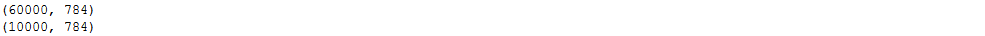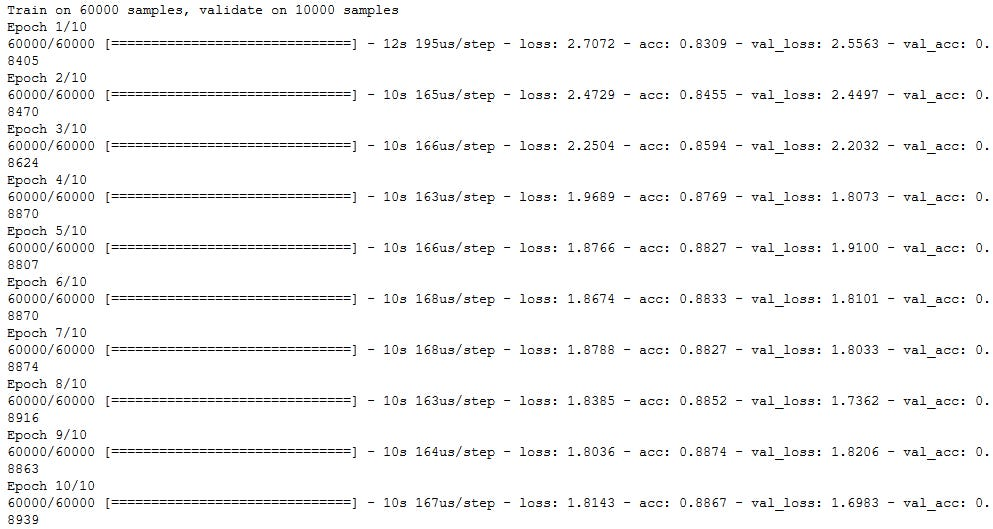# Keras Introduction

This article was originally published on my website.

In this article we will go over the basics of Keras. We will discuss what Keras is, why we should use it as well as the steps we can take to build an simple image classifier.

### What is Keras?

Keras is an open-source high-level neural network library written in Python. It is capable of running on top of TensorFlow, CNTK and Theano.

### Why use Keras?

Keras is an excellent tool for deep learning especially for beginners. It’s main focus is enabling fast experimentation using easy to understand and clear syntax. Because it runs on top of Tensorflow or Theano you can train on both CPU and GPU without problems.

### Installing Keras

For installation you can use the Python Package Manager(pip) or you can clone the github repsitory and install that way.

pip install:

``pip install keras``

install using git

``git clone https://github.com/keras-team/keras.git``
``cd kerassudo python setup.py install``

### Load in dataset

The Keras libary has a few datasets which you only need to import like the mnist or cifar100 dataset. We can load them in using the keras.datasets module.

``from keras.datasets import mnist``
``(x_train, y_train), (x_test, y_test) = mnist.load_data()``

### Create a model

In Keras we can use to different methods for creating a model. The Sequential model API is the easier method but it doesn’t include as much possibilities as the Model API.

For this article we will create a simple feed forward neural network using the sequential api.

Importing Sequential api and layers.

``from keras.models import Sequentialfrom keras.layers import Dense``

In Keras you create a model by adding one layer after the other.

``model = Sequential()model.add(Dense(256, activation='relu'))model.add(Dense(10, activation='softmax'))``

### Compile model

Now that we have defined our model we can compile it.

When compiling we must specify a few import parameters. Mainly the loss function and the optimizer. We can also specify metrics which we can then see in the training process.

When we compile the backend libary we are using (Tensorflow or Theano) is choosing the best way to train our model on the given hardware.

``model.compile(loss='binary_crossentropy', optimizer='adam', metrics=['accuracy'])``

### Fit model on training data

We have defined our model and compiled it so now we are ready to fit our model on the training dataset.

To fit our model we use the fit() method. We need to pass it our X and Y data as well as the number of epochs and the batch size.

Epochs are the number of times we train over the whole dataset and the batch size specifies how many training examples we will load into memory at once.

We can also pass it an validation_dataset so we can see the performance on both training and testing set.

Preprocessing data:

``from keras.utils import to_categoricaly_train = to_categorical(y_train, num_classes=10)y_test = to_categorical(y_test, num_classes=10)x_train = x_train.reshape(len(x_train), 28*28)x_test = x_test.reshape(len(x_test), 28*28)print(x_train.shape)print(x_test.shape)``Data Dimensionality

Now that we have preprocessed our data we can fit our model. We will save the results so we can visualize them in the next step.

``history = model.fit(x_train, y_train, epochs=10, batch_size=32, validation_data=(x_test, y_test))``Training Information

As you can see we are getting pretty bad results and that is because of two main reasons. First of we aren’t training a lot of epochs and secondly the model structure is really basic. You can get better result by implementing a convolutional neural network(CNN). If you are interested you can check out my video on CNNs in Keras here.

### Visualizing the training process.

We can visualize our training and testing accuracy and loss for each epoch so we can get intuition about if our model is overfitting or underfitting. We can get the accuracy and loss data from the history variable. We will use Matplotlib to graph it.

``import matplotlib.pyplot as pltplt.plot(history.history['acc'], label='training accuracy')plt.plot(history.history['val_acc'], label='testing accuracy')plt.title('Accuracy')plt.xlabel('epochs')plt.ylabel('accuracy')plt.legend()``
``plt.plot(history.history['loss'], label='training loss')plt.plot(history.history['val_loss'], label='testing loss')plt.title('Loss')plt.xlabel('epochs')plt.ylabel('loss')plt.legend()``

In the graphs above we can see that our model isn’t overfitting as well as that we could train more epochs because the validation loss is still decreasing.

### Summary

In this article, we went over what Keras is. How to install it as well as a step by step guide on how to use it including:

• Loading in Dataset
• Creating a model
• Compiling the model
• Training the model
• Visualizing the training process

If you liked this article consider subscribing on my Youtube Channel and following me on social media.

If you have any questions fell free to dm me on Twitter or leave a comment.

Source: Deep Learning on Medium